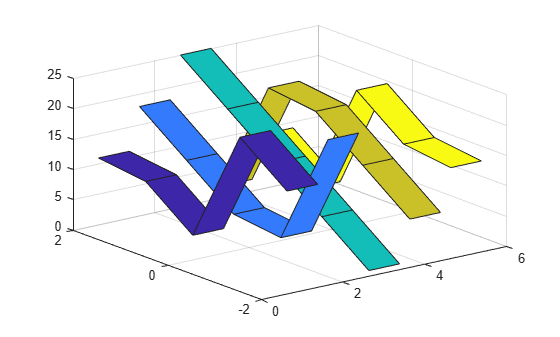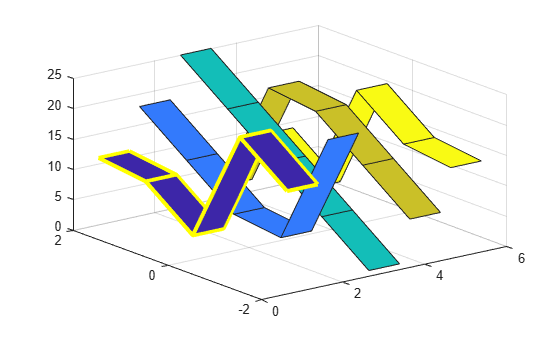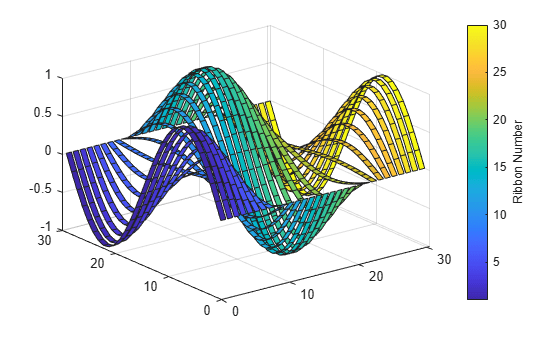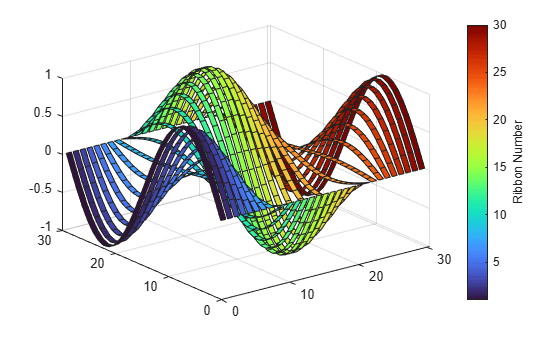# ribbon

•## Syntax

``ribbon(Z)``
``ribbon(Y,Z)``
``ribbon(Y,Z,width)``
``ribbon(ax,___)``
``s = ribbon(___)``

## Description

example

````ribbon(Z)` plots the columns of `Z` as three-dimensional ribbons of uniform width, where y-coordinates range from 1 to the number of rows in `Z`. Ribbons advance along the x-axis centered at unit intervals. ```

example

````ribbon(Y,Z)` plots three-dimensional ribbons at the locations specified by `Y`.```

example

````ribbon(Y,Z,width)` specifies the width of the ribbons.```
````ribbon(ax,___)` plots into the axes specified by `ax` instead of the current axes (`gca`). The `ax` option can precede any of the input argument combinations in the previous syntaxes.```

example

````s = ribbon(___)` returns a vector of `Surface` objects with one object per ribbon. Use `s` to modify properties of the plot after creating it. For a list of properties, see Surface Properties.```

## Examples

collapse all

Create a plot with five ribbons at increasing heights. First, create a 5-by-5 matrix with elements corresponding to ribbon heights.

`Z = repmat(1:5,4,1)`
```Z = 4×5 1 2 3 4 5 1 2 3 4 5 1 2 3 4 5 1 2 3 4 5 ```

Each column of `Z` represents one ribbon, plotted at a constant x-coordinate corresponding to the column number and with y-coordinates corresponding to the row numbers of `Z`.

`ribbon(Z)`Create a 5-by-5 matrix with the `magic` function.

`Z = magic(5)`
```Z = 5×5 17 24 1 8 15 23 5 7 14 16 4 6 13 20 22 10 12 19 21 3 11 18 25 2 9 ```

Create a ribbon plot of the matrix and specify the y-coordinates so each ribbon is centered at 0.

```Y = [-2 -1 0 1 2]; ribbon(Y,Z);```Plot three ribbons at different locations along the y-axis. Specify the y-coordinates of the ribbons as a matrix `Y` that is the same size as `Z`, the matrix of ribbon heights. Each column of `Y` corresponds to one ribbon.

```Y = [1 2 3; 2 3 4; 3 4 5; 4 5 6]; Z = Y; ribbon(Y,Z)```Create a ribbon plot and set the width of each ribbon to 30% of the total space available.

```Z = magic(5); Y = [-2 -1 0 1 2]; ribbon(Y,Z,0.3)```Create a ribbon plot and specify an output argument. The output is a vector of five `Surface` objects, where each object corresponds to one ribbon.

```Z = magic(5); Y = [-2 -1 0 1 2]; s = ribbon(Y,Z)``````s = 5x1 Surface array: Surface Surface Surface Surface Surface ```

Highlight the first ribbon by changing the `EdgeColor` and `LineWidth` properties of the corresponding `Surface` object.

```s(1).EdgeColor = "yellow"; s(1).LineWidth = 3;```Create a ribbon plot with 30 ribbons and a colorbar.

```t = linspace(0,2*pi,30); x = sin(t)'; y = cos(t); ribbon(x*y) cbar = colorbar; cbar.Label.String= "Ribbon Number";```Change the ribbon colors using the `colormap` function. `ribbon` maps the x-coordinates of the ribbons to colors in the colormap linearly.

`colormap(turbo)`## Input Arguments

collapse all

z-coordinates that represent ribbon heights, specified as a numeric vector or numeric matrix.

• If `Z` is a vector, `ribbon` creates a single ribbon regardless of whether `Z` is a row or column vector.

• If `Z` is a matrix, `ribbon` creates one ribbon for each column. Ribbons advance along the x-axis centered at unit intervals, where x-coordinates range from 1 to the number of columns in `Z`.

y-coordinates, specified as a numeric vector or numeric matrix. The size of `Z` determines the possible sizes of `Y`:

• If `Z` is a vector, `Y` must be a vector of the same size as `Z`. `ribbon` plots a single ribbon at `X = 1` using the data in `Y` and `Z`.

• If `Z` is a matrix, `Y` can be a row or column vector with a length equal to the number of rows in `Z`, or a matrix of the same size as `Z`. `ribbon` plots a ribbon for each column of `Z` using the data in `Y` and `Z`. If `Y` is a vector, each ribbon has the same y-coordinate.

Ribbon width, specified as a numeric scalar representing a percentage of the total space available for each ribbon.

• If `width < 1`, the ribbon width occupies that fraction of the allocated space.

• If `width = 1`, the ribbons touch one another, leaving no space between them when viewed down the z-axis.

• If `width > 1`, the ribbons overlap and can intersect.

For example, the default value of `0.75` means the ribbon width is 75% of the total space available for the ribbon, with 12.5% of empty space on each side.

Target axes, specified as an `Axes` object. If you do not specify the axes, MATLAB® plots into the current axes or it creates an `Axes` object if one does not exist.

## Version History

Introduced before R2006a